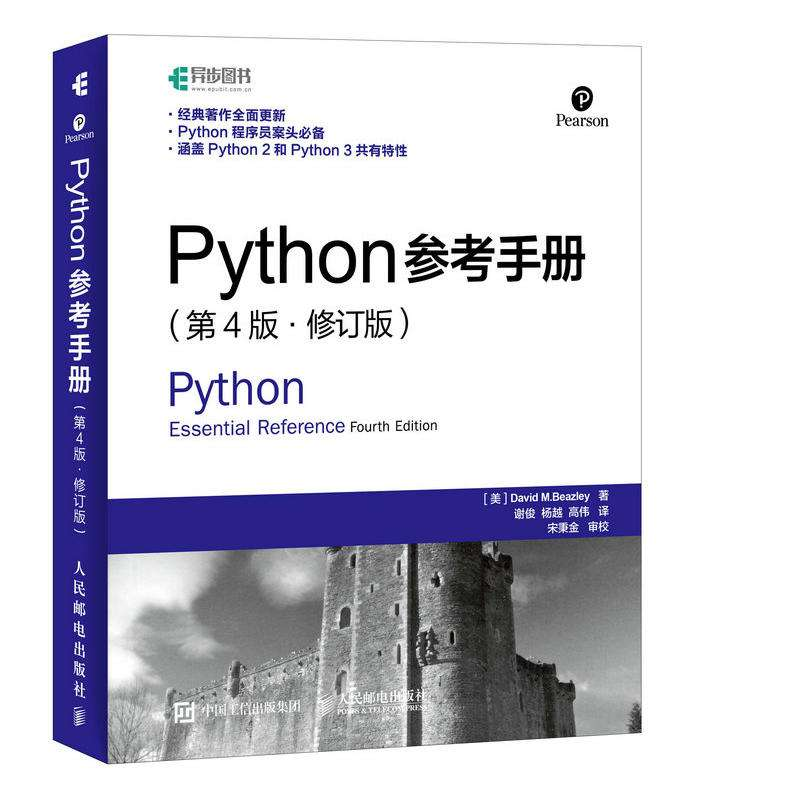# Python 对象引用与复制 (Python参考手册读书笔记)

Python 对象引用与复制 (Python参考手册读书笔记) ![Python参考手册读书笔记](## 通过赋值创建一个副本

``````a = [1,2,3,4,5]
b = a
print(b is a )
b = -99
print(a)
``````

### 浅复制 通过结果可以发现一些不同

``````c = [11,22,]
d = list(c)
print(c is d)
d.append(33)
print(c)
print(d)
d = 00
print(c)
print(d)
``````

### 深复制

``````import copy
e = [11,12,13]
f = copy.deepcopy(e)
print(e is f)
f = 78
print(e)
print(f)
``````